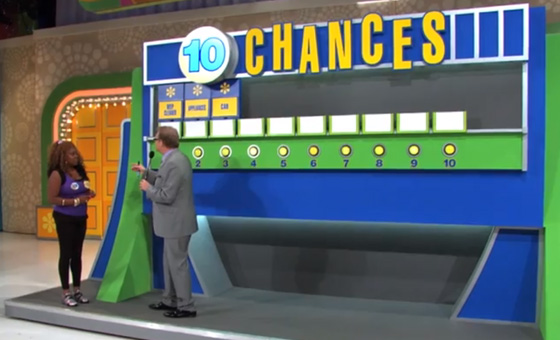Ten Chances Photos & VideoVIDEO: Ten Chances
Game Description The contestant is given a total of ten chances to write down the prices of a two-digit prize, a three-digit prize, and a car. The contestant is shown three digits, two of which are the price of the two-digit prize. Once that is won, the contestant is shown four digits, three of which are the price of the three-digit prize. If that is won, then the contestant is shown the five digits in the price of the car.